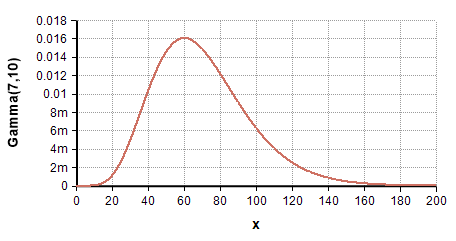# Gamma distribution

(Redirected from Gamma)

The Gamma distribution is a continuous, positive-only, unimodal distribution that encodes the time required for «alpha» events to occur in a Poisson process with mean arrival time of «beta»Use the Gamma distribution with «alpha» > 1 if you have a sharp lower bound of zero but no sharp upper bound, a single mode, and a positive skew. The LogNormal distribution is also an option in this case. Gamma() is especially appropriate when encoding arrival times for sets of events. A gamma distribution with a large value for «alpha» is also useful when you wish to use a bell-shaped curve for a positive-only quantity.

The gamma distribution is bounded below by zero (all sample points are positive) and is unbounded from above. It has a theoretical mean of alpha*beta and a theoretical variance of alpha*beta^2. When «alpha» > 1, the distribution is unimodal with the mode at (alpha - 1)*beta. An exponential distribution results when alpha = 1. As $\displaystyle{ \alpha \to \infty }$ , the gamma distribution approaches a normal distribution in shape.

## Functions

Note

Some textbooks use Rate = 1/beta, instead of «beta», as the scale parameter.

### Gamma(alpha, beta, over)

The distribution function. Use this to describe a quantity that is gamma-distributed with shape parameter «alpha» and scale parameter «beta». The scale parameter, «beta», is optional and defaults to beta = 1.

### Dens_Gamma(x, alpha, beta)

To use this, you need to add the Distribution Densities Library to your model.

The analytic probability density of the Gamma distribution at «x». Returns

$\displaystyle{ p(x) = {{\beta^{-\alpha} x^{\alpha-1} \exp(-x/\beta)}\over{\Gamma(\alpha)}} }$

### CumGamma(x, alpha, beta)

To use this, you need to add the Distribution Densities Library to your model, or use GammaI instead.

The cumulative density up to «x», given for $\displaystyle{ x\gt 0 }$ by

$\displaystyle{ F(x) = {1\over {\Gamma(\alpha)}} \int_0^x \beta^{-\alpha} t^{\alpha-1} \exp(-t/\beta) dt }$

This is also the same as the regularized incomplete gamma function, computed by the function GammaI.

### CumGammaInv(p, alpha, beta)

To use this, you need to add the Distribution Densities Library to your model, or use GammaIInv instead.

The analytic inverse cumulative probability function (quantile function). Returns the pth fractile/quantile/percentile for the gamma distribution. Same as the inverse incomplete gamma function, GammaIInv.

## When to use

Use the Gamma distribution with «alpha» > 1 if you have a sharp lower bound of zero but no sharp upper bound, a single mode, and a positive skew. The LogNormal distribution is also an option in this case. Gamma() is especially appropriate when encoding arrival times for sets of events. A gamma distribution with a large value for «alpha» is also useful when you wish to use a bell-shaped curve for a positive-only quantity.

## Statistics

The theoretical statistics (i.e., in the absence of sampling error) for the gamma distribution are as follows.

• $\displaystyle{ 0\lt x\lt \infty }$
• $\displaystyle{ \alpha, \beta\gt 0 }$
• Mean = $\displaystyle{ \alpha \beta }$
• Mode = $\displaystyle{ Max([0, \alpha-1) \beta]) }$
• Variance = $\displaystyle{ \alpha \beta^2 }$
• SDeviation = $\displaystyle{ \beta \sqrt{\alpha} }$
• Skewness = $\displaystyle{ 2 / \sqrt{\alpha} }$
• Kurtosis = $\displaystyle{ 6 / \alpha }$

## Parameter Estimation

Suppose X contains sampled historical data indexed by I. To estimate the parameters of the gamma distribution that best fits this sampled data, the following parameter estimation formulae can be used:

alpha := Mean(X, I)^2/Variance(X, I)
beta := Variance(X, I)/Mean(X, I)

The above is not the maximum likelihood parameter estimation, which turns out to be rather complex (see Wikipedia). However, in practice the above estimation formula perform excellently and are so convenient that more complicated methods are hardly justified.

The Gamma distribution with an «offset» has the form:

Gamma(alpha, beta) - offset

To estimate all three parameters, the following heuristic estimation can be used:

alpha := 4/Skewness(X, I)^2
offset := Mean(X, I) - SDeviation(X, I)*Sqrt(alpha)
beta := Variance(X, I)/(Mean(X, I) - offset)